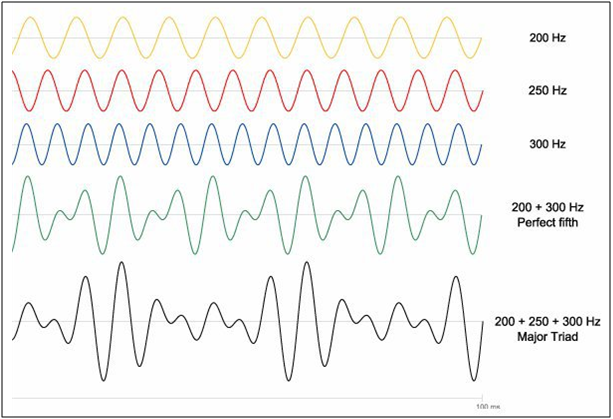## Maths & Music Relationship

Have you ever wondered the realtionship between maths & music?

Both art and mathematics are disciplines that seek to understand and create patterns. The art of music, specifically, relies heavily on the use of mathematical patterns for its progression and development over the years. Music is created using the fundamental rules of scales, chords, and harmonies, which are derived directly from the different mathematical relationships between the frequencies of musical notes. The major and minor scales, as we know them today, were devised in the Baroque era. During this period, advances in mathematics also enabled the extensive development of music, which helped renowned composers like Johann Sebastian Bach to compose in any musical scale, as compared to the single scale used before.

#### Maths & music

These scales were derived by dividing an octave into equal frequency ratios. An octave is known as the distance between any two musical notes where the second note has exactly twice the frequency of the first. For example, if C3 has a frequency of approximately 130.81 Hz, then C4 (which is an octave above it) would have double the frequency when played. This is, in fact, true, since the frequency of C4 is 261.62 Hz! In modern western classical music, an octave is divided into 12 steps, each of which is a “semitone” away from its predecessor. To equally divide an octave into 12 steps, such that the last note of the octave would be exactly double the frequency of the first, musicians were required to calculate the twelfth root of two ( ). This was an especially difficult task, considering that there were no modern-day calculators to help with it. However, advances in mathematics, during the Baroque period, allowed mathematicians to easily calculate , which therefore enabled musicians to devise the 12 notes that comprised any octave.

Hence, the frequency of each successive semitone is  times the frequency of its predecessor. For example, if C3 has a frequency of 130.81 Hz, then the frequency of C#3 would be  This is true, since the actual frequency of C#3 is approximately 138.59 Hz! Similarly, if a note is 7 semitones away from the root note (an interval that is more commonly known as the perfect fifth in music theory), the frequency of that note would be , which is approximately 196.00 Hz. This is, in fact, the actual frequency of G3, which is the perfect fifth interval of the C3 scale!

Therefore, to understand this in a more simplified way, the frequencies of the 12 notes of a scale can be devised by using the following formula:

Where F is the frequency of the root note, and n is the number of semitones of each note as we move away from the root note. Major and minor scales can then be derived from this by using the tone-semitone formulas. This is the exact same development that allowed Bach to write The Well-Tempered Clavier(1722)in all 24 major and minor keys. Before this, music was not known to really modulate in different keys.

Another interesting frequency relationship can be graphically noticed in theintervals that make up what is commonly known as a major chord. The major chord, one of the most popular chords of music, is played by combining the following intervals: the root note, the major third, and the perfect fifth. As shown by the diagram below, each of these intervals is related to each other by an approximate frequency ratio of 4:5:6. This means that the waves meet at every 4 wavelengths of the 200 Hz wave, every 5 wavelengths of the 250 Hz wave, and every 6 wavelengths of the 300 Hz wave.

These waves
superimpose upon each other to produce the complex waveform as shown by the 200
+ 250 + 300 Hz Major Triad
waveform below. This periodic intersection of
each of the waves is what produces a sound that is pleasant to the ears and is
known as consonanceThis mathematical relationship between the notes is also what enabled Ludwig van Beethoven to compose one of the most renowned pieces of classical music – Moonlight Sonata, even as he was slowly going deaf. Contrasting the consonance displayed by the above notes with dissonance, he was able to bring out the very emotions that give Moonlight Sonata it’s prominently praised reputation.

To know more about the interesting ways in which music is heavily reliant on mathematics, the following links may also be visited:

·        “Music and Math. The Genius of Beethoven.”  https://www.youtube.com/watch?v=M51Bm9sNazM

·        “Combining Math And Music”https://www.uchicago.edu/features/combining_math_and_music/